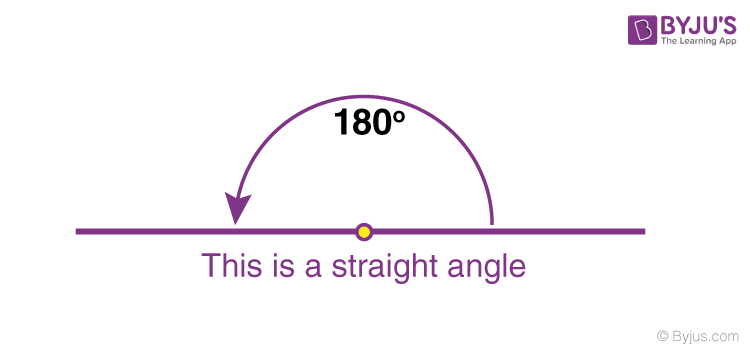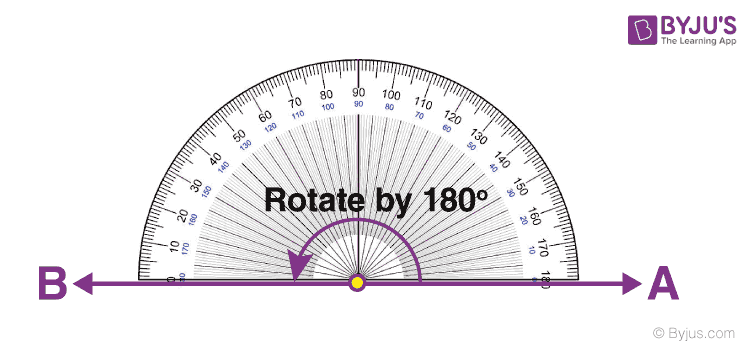# Straight Angle

In Maths, a straight angle is an angle equal to 180 degrees. It is called straight because it appears as a straight line. Basically, as per angles definition in maths, when two rays are joined end to end, they form an angle. Hence, the two rays of the 180-degree angle are subtended towards the opposite direction, where the rays are joined to each other at endpoints.

In radians, the 180-degree angle is measured in pi (π). To point the opposite way, the straight angle changes the direction. A 180 degree is also considered as supplementary. Apart from this angle, there are five different types of angles in geometry which you can learn here.

 Facts: A straight angle always forms a straight line. The value of the straight angle is 180°. The arms of the straight angle lie opposite to each other from the vertex point. The straight angle measured anticlockwise is the positive straight angle i.e.180°. The straight angle measured clockwise is a negative straight angle i.e.-180°.

## Straight Angle Definition

In geometry, a straight angle is an angle, whose vertex point has a value of 180 degrees. Basically, it forms a straight line, whose sides lie in opposite directions from the vertex. It is also termed as “flat angles”A horizontal line and a vertical line are always straight lines and therefore they are examples of straight angles.

### Notation

In degrees, the straight angle is represented as 180° and in radians, it is denoted with pi (π).

### Straight Angle Examples

Some of the straight angle examples in our day-to-day life are:

• A flat surface has an angle of 180 degrees
• A straight stick has an angle which is straight or 180 degree
• A plane inclined staircase represents a straight angle
• Angle formed in a see-saw

A straight angle is different from the straight line, as it measures 180 degrees and a straight line is a connector of two points.

## Theorem

Straight angle theorem states that all the straight angles are 180 degrees. If the legs of angle, are pointing exactly in the opposite directions, then it forms a straight angle. A straight angle is represented as 180° (in degrees) or π (in radians).

One good example is the line segment in geometry, the endpoints of which extends towards the opposite direction.

## Properties of Straight Angles

Some important properties of straight angles are as follows:

• A straight angle measures exactly half of a revolution
• A straight angle is produced by revolving one ray by 180° with respect to another ray
• In a straight angle, the arms extend in the opposite directions
• A straight angle modifies the direction of a point
• A straight angle can also be formed by joining two right angles

## Six Types of Angles

An angle is formed when two line segments are joined at a single endpoint or when two line segments are combined at a single endpoint. The point where the two lines meet is called the Vertex of the angle. There are basically 6 different types of angles whose vertex values are different, respectively, and they are;

• Acute Angle (less than 90°)
• Obtuse Angle (more than 90°)
• Right Angle (equal to 90°)
• Straight Angle (exact 180°)
• Reflex Angle (greater than 180°) and
• Full Angle (Exact 360°)

An angle with 0° is called a zero angle, means it doesn’t form an angle. These are the general classification of angles.

In geometry, we compare two angles based upon their conditions. Some angles are defined in terms of their similarity, called as a congruent angle, which has the same angle. Then, we have adjacent angles, which are adjacent to each other. Complementary angles, whose sum is equal to 90°.

## Can we consider a Triangle Made from a Straight Angle?

A straight angle has a measure of 180°and the sum of interior angles of a triangle is also 180°. But we cannot consider straight angle as a straight angle triangle because, for a triangle, the figure should form an enclosed structure.

Triangle is a closed three-sided figure, which has three distinct points which are not collinear with each other.  But, in the case of the Straight line, the points are collinear and hence they cannot form a closed structure. Therefore, there is no triangle formed.

## How to construct a Straight Angle?

The steps to construct a Straight Angle, are as follows:

• Draw a straight-line OB using a ruler, with an arrowhead at B
• Now place the protractor on this line such that the baseline of the protractor is adjusted over OB. The point B should be facing towards the 0°of the protractor.
• Now mark the point, starting from 0° and moving towards 180 degrees. On reaching 180°, mark a point A on the paper.
• Now, join the vertex of the line OB with the marked point A. The second ray OA should have arrowhead at A.Q1

### What is called Straight angle?

A straight angle is an angle equal to 180 degrees. It looks like a straight line. In radian, it is measured as π angle.
Q2

### What is the Angle of a Straight Angle?

The angle of a straight angle is 180°.

Q3

### What is Half of a Straight Angle?

The half of a straight angle is a right angle. Since a straight angle is 180 degrees, the half of it will be 90 degrees which are a right angle.

Q4

### Is a straight line a reflex angle?

No, a straight line is a straight angle and not a reflex angle. It should be noted that a reflex angle measures more than 180° and less than 360°.

Q5

### Is a straight line a straight angle?

A straight angle with two endpoints can be considered to be a straight line.
Q6

### What are the other five types of angles?

The other types of angles apart from straight angle are:
Acute angle, Obtuse angle, Right angle, Reflex angle and Full Angle
Q7

### How to form a straight angle?

A straight angle can be formed by adding two right angle or two 90 degrees.
90 + 90 = 180 degrees
Q8

### Can we make triangle using straight angle?

No, we cannot make triangle using a straight angle, because straight angle is itself equal to 180 degrees, whereas the total of all three angles of a triangle is equal to 180 degrees.
Q9

### Can straight angle be negative?

The straight angle measured clockwise is a negative straight angle i.e.-180°.